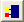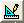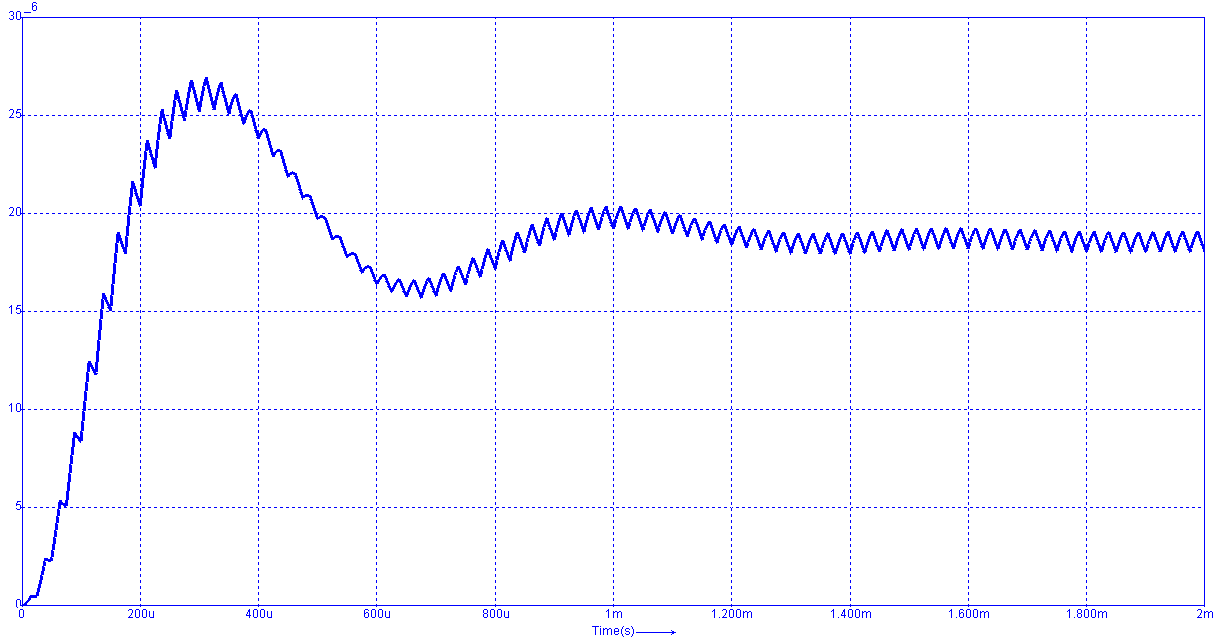## Set up a switched mode power supply simulation (Boost converter)

In the first four tutorials, we already practiced how to model a boost converter with a solar system. In this tutorial, we will try to build a voltage mode PWM controller by using a boost converter. The example we�re going to use is seems as general sample on boost converter modeling which is required for maximum power point control of solar cell as below. The upper part of the schematic is a voltage source, a boost converter and its load; the lower part is a PWM controller which senses the voltage and compares it in order to controls the switch (the MosfetLevel0) of the boost converter. Hence in this schematic, we have four parts as below: Boost converter, load, error amplifier which senses the voltage from load, and the comparator which sends the PWM control signal to the switch.To build the above schematic, the necessary components are as below. To select the components from component list, find out the location of the necessary component, left-click the component and release the mouse button, and then move your mouse cursor into the workscreen and left-click again. The parameters of each component are shown in brackets behind. Right-click components for editing the required parameters.

Using components:

• Components/Circuit/Sources/V(V2: Name=v2, value=10; Vref: Name=VREF, value=5)
• Components/Circuit/Sources/VTRIANGLE(Name=Vosc, Model Parameters: DC=5, AC=6, F[Hz]=40k, Phase[Degrees]=0, d[0..1]=1)
• Components/Circuit/RLC/R(Rgate: Name=Rgate, value=10; Resr: Name=Resr, value=10m; RL: Name=RL, value=20; RS1: Name=RS1, value=300k; RS2: Name=RS2, value=100k; R4: Name=R4, value=20; R2: Name=R2, value=10k; R1: Name=R1, value=1k)
• Components/Circuit/RLC/L(L1: value=100u, initial condition=0)
• Components/Circuit/RLC/C(C1: value=22u, initial condition=0)
• Components/Library/Semiconductor/Mosfet/MosfetLevel0(BV=1e6, CGS=1nF, RG=10, RoffDiode=1e9, RoffMosfet=1e9, RonDiode=10m, RonMosfet=100m, VTO[V]=3, VonDiode=0.6)
• Components/Library/Semiconductor/OpAmp/Comperator(DCGain=100k, Rin=2meg, Ro=75)
• Components/Library/Semiconductor/OpAmp/OpAmp(Cin=14p, DCGain=100k, Rin=2meg, Ro=75, SlewRate[V/s]=6e6, UnityBandWidth=1meg)
• Components/Library/Electric/Ground/Ground
• Components/Library/Sensor/Voltage/v(Gain=1)

We can find the above components in the following paths:• Assign a label: (Click with the right mouse button on the node. Refer to Introduction/ Simple Circuit to know how to use �label�.)
• The anode of V3 � V_3
• The anode of R1 � V_1
• The output of OpAmp � V_2
• The anode of RGATE � gate• Components/Blocks/Sinks/SCOPE or Insert/Scope or click the short-cut buttonin the experiment bar
• (Scope2: read the value of V_3 to the first input trace; Scope4: assign the current value of L1 to the first input trace and the current value of D1 to the second input trace; Scope3: read the values of V1 and V2 to the first two input traces and gate to the third input trace.)
• (Notice that in the previous tutorial (application/ LED current control), we used a resistor to change dot of Scope to round. In this tutorial, we can also use the same method or use a voltage sensor to change the circuit node to signal node. Even for current value, we can also use a current sensor to change circuit node to signal node.)Simulation parameters: (Go to Simulation/Simulation Parameters or click the short-cut buttonin the menu bar)

• Screen width (Tscreen) =2m
• Integration step size (dt)=100n
• Show each 1 timestep simulation results
• Select: Wait After Screen
• Numerical Integration Method Circuit: Euler (To know the differences between the three methods, refer to Introduction/ Simulation Parameters)

After configuring all the necessary parameters, go to Simulation/Start simulation to execute the simulation or click the short-cut buttonin the menu bar and get the following result.The output voltage of the boost converter: V_3The blue curve stands for the current value of the inductor L1, and the red curve for the current value of the diode D1. We can see the current value of D1 depends on the on or off of the switch which is controlled by the PWM controller.The blue curve stands for the feedback voltage V_1. The red curve stands for the voltage which is compared with the reference voltage. And the sky blue curve is the calculated signal from comparator and used for controlling the switch.